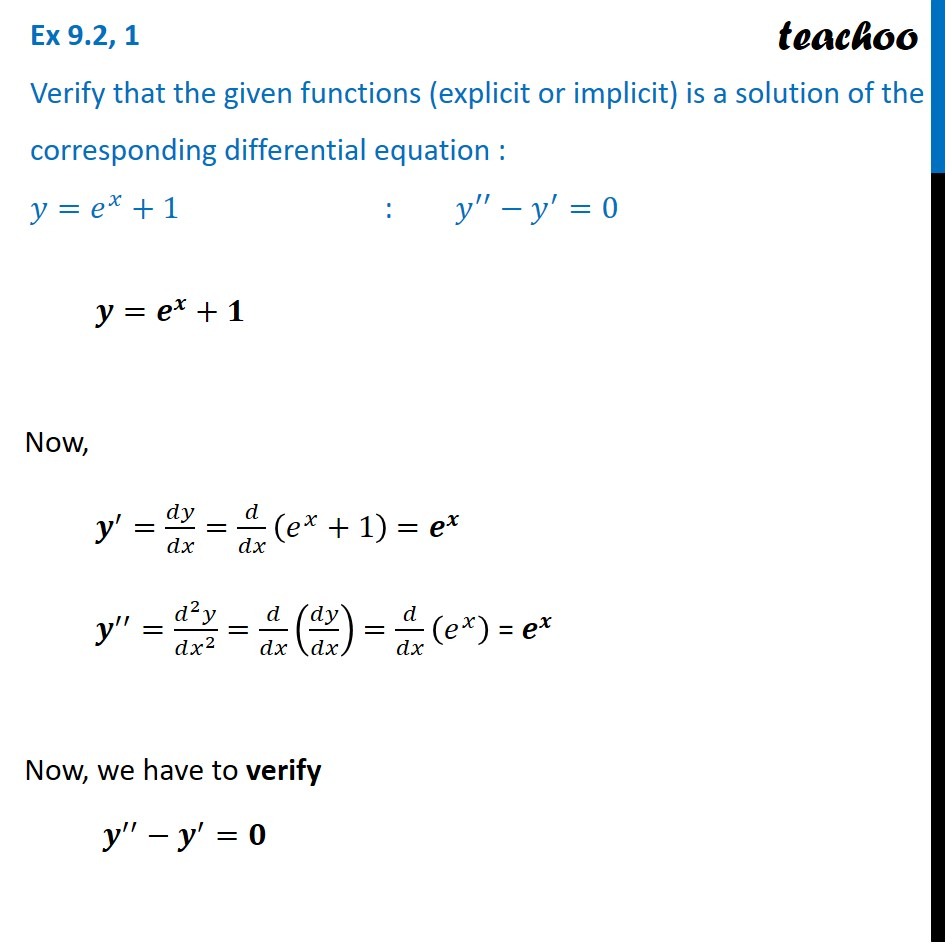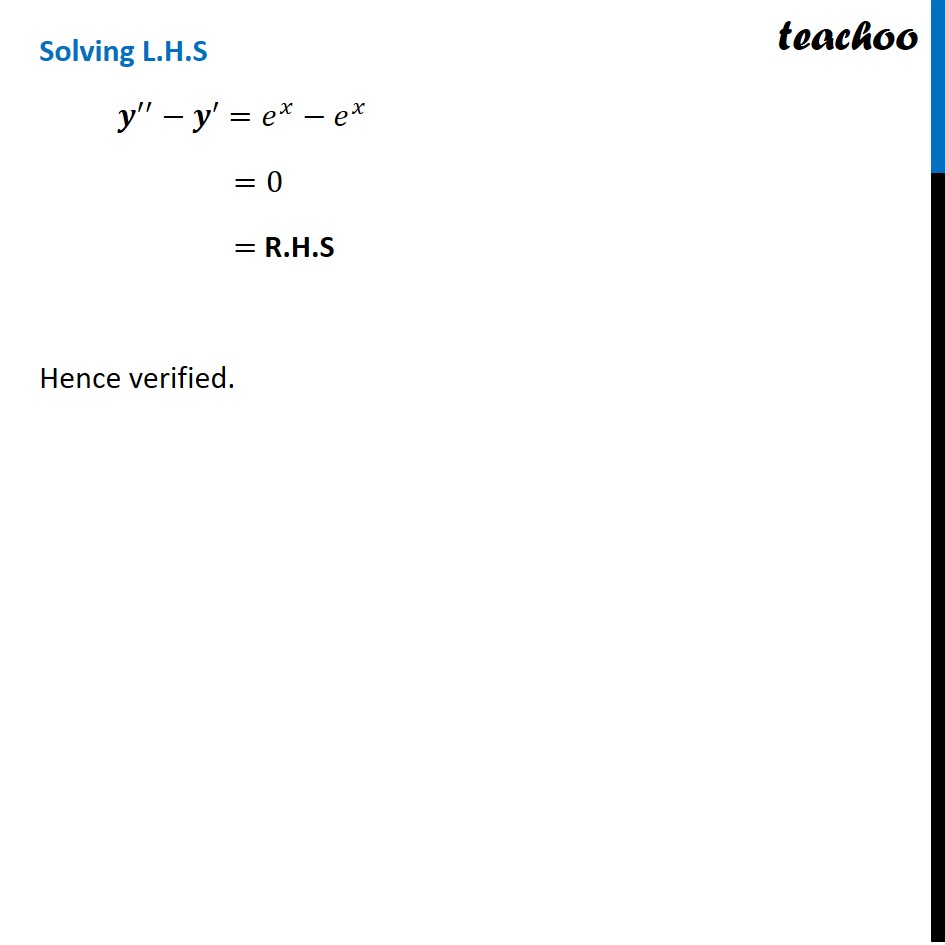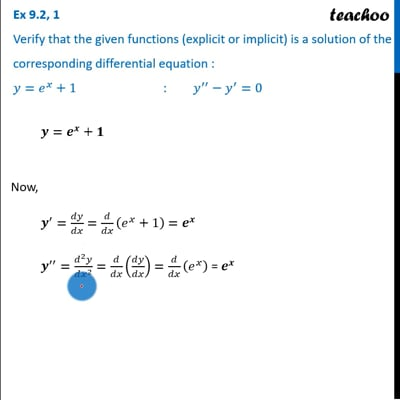Ex 9.2

Chapter 9 Class 12 Differential Equations
Serial order wiseThis video is only available for Teachoo black usersThis video is only available for Teachoo black users

Learn in your speed, with individual attention - Teachoo Maths 1-on-1 Class

### Transcript

Ex 9.2, 1 Verify that the given functions (explicit or implicit) is a solution of the corresponding differential equation : 𝑦=𝑒^𝑥+1 : 𝑦^′′−𝑦^′=0 𝒚=𝒆^𝒙+𝟏 Now, 𝒚^′=𝑑𝑦/𝑑𝑥=𝑑/𝑑𝑥Solving L.H.S 𝒚^′′−𝒚^′ =𝑒^𝑥−𝑒^𝑥 =0 = R.H.S Hence verified. Solving L.H.S 𝒚^′′−𝒚^′ =𝑒^𝑥−𝑒^𝑥 =0 = R.H.S Hence verified.